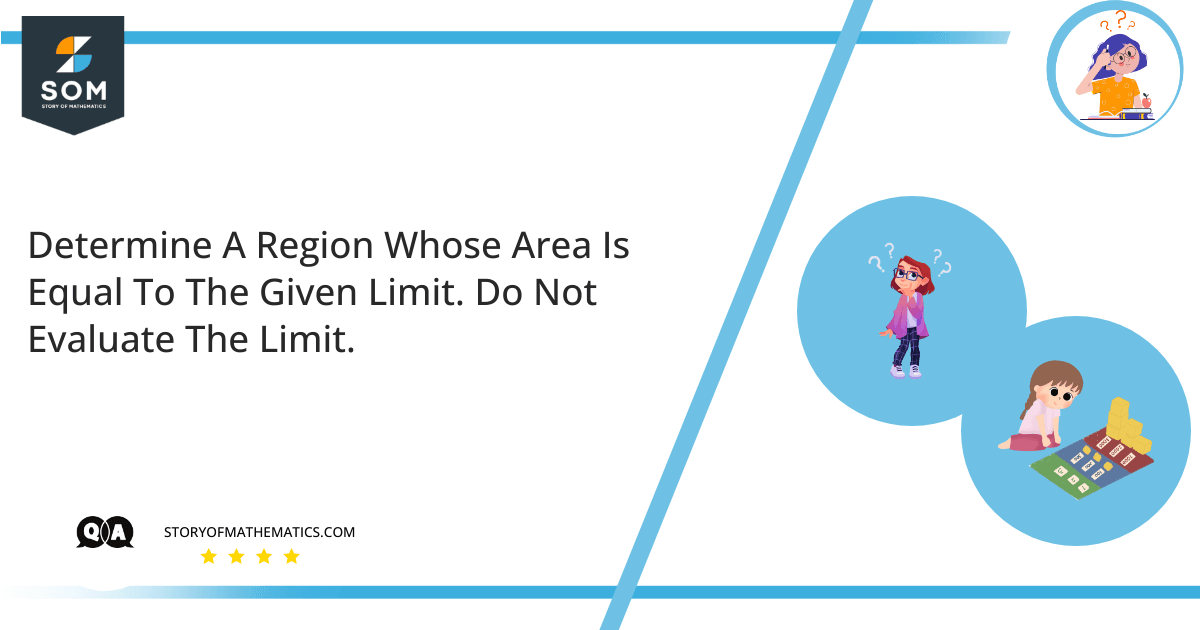# Determine a region whose area is equal to the given limit. Do not evaluate the limit.$\lim_{n\to\infty}\sum_{i=1}^{n}\frac{\pi}{4n}{tan\left(\frac{i\pi}{4n}\right)}$

The basic concept behind this guide is the use of the Limit Function to determine an area of the region. The area of a region that covered the space above the $x-axis$ and the below the curve of given function $f$ integrable on $a$ to $b$ is calculated by integrating the curve function over a limit interval. The function is expressed as follows:

$\int_{a}^{b}{f(x)dx}$

The area of the region enclosed by $x-axis$ and curve function $f$ is expressed in limit form as follows:

$\int_{a}^{b}{f(x)dx}=\lim_{n\to\infty}\sum_{i=1}^{n}f{(x_i)}∆x$

Where:

$x_i=a+i ∆x$

So:

$\int_{a}^{b}{f(x)\ dx}\ =\lim_{n\to\infty}\sum_{i=1}^{n} f(a+i∆x) ∆x$

Here:

$∆x = \frac{b-a}{n}$

Given Function is:

$\int_{a}^{b}{\ f(x)\ \ dx}\ =\ \lim_{n\to\infty} \sum_{i\ =\ 1}^{n}{\ \frac{\pi}{4n}}{\ tan\ \left(\frac{i\pi}{4n}\right)}$

We know that the standard form for an area of the region:

$\int_{a}^{b}{f(x)\ dx}\ =\lim_{n\to\infty}\sum_{i=1}^{n} f(a+i∆x) ∆x$

Comparing the given function with the standard function, we find the value of each component as follows:

$a\ +\ i\ ∆x = \frac{i\pi}{4n}$

Hence:

$a\ =\ 0$

$∆x = \frac{\pi}{4n}$

As we know:

$∆x = \frac{b-a}{n}=\frac{\pi}{4n}$

$\frac{b-0}{n}\ =\ \frac{\pi}{4n}$

$b\ =\ \frac{\pi}{4}$

Let’s consider:

$f(x)\ =\ tan\ (x)$

So:

$\lim_{n\to\infty}\sum_{i=1}^{n}\frac{\pi}{4n}{tan\left(\frac{i\pi}{4n}\right)}\ =\ \int_{a}^{b}{\ f(x)\ dx}$

Substituting the values on the left-hand side of the above expression:

$\lim_{n\to\infty}\sum_{i=1}^{n}\frac{\pi}{4n}{tan\left(\frac{i\pi}{4n}\right)}\ =\ \int_{0}^{\frac{\pi}{4}}{\ tan\ (x)\ dx\ =\ 0.346}$

The equation for the curve is:

$f(x)\ =\ tan\ (x)$

The interval for $x-axis$ is:

$x\ \in\ \left[0,\ \frac{\pi}{4}\right]$

It is represented by the following graph:Figure 1

## Numerical Result

The region, having an area defined by the given limit, is equal to the region below the following curve function and above $x-axis$ for the given interval, as follows:

$f(x)\ =\ tan(x),\ \ x\ \in\ \left[0,\ \frac{\pi}{4}\right]$Figure 1

## Example

Find an expression for the region having an area equal to the following limit:

$\lim_{n\to\infty}\ \sum_{i\ =\ 1}^{n}{\ \frac{2}{n}}\ {\left(5\ +\ \frac{2i}{n}\right)}$

Solution

Given Function is:

$\int_{a}^{b}{\ f(x)\ dx}\ =\ \lim_{n\to\infty}\ \sum_{i\ =\ 1}^{n}{\ \frac{2}{n}}{\ \left(5\ +\ \frac{2i}{n}\right)}$

We know that the standard form for an area of the region:

$\int_{a}^{b}{f(x)\ dx}\ =\lim_{n\to\infty}\sum_{i=1}^{n} f(a+i∆x) ∆x$

Comparing the given function with the standard function, we find the value of each component as follows:

$a\ +\ i∆x = 5 + i \frac{2}{n}$

Hence:

$a\ =\ 5$

$∆x =\frac{2}{n}$

As we know:

$∆x = \frac{b-a}{n}$

$\frac{b-5}{n}\ =\ \frac{2}{n}$

$b\ =\ 7$

Let’s consider:

$f(x)\ =\ 5\ +\ x$

So:

$\lim_{n\to\infty}\ \sum_{i\ =\ 1}^{n}{\ \frac{2}{n}}\ {\left(5\ +\ \frac{2i}{n}\right)}\ =\ \int_{a}^{b}{\ f(x)\ dx}$

Substituting the values on the left-hand side of the above expression:

$\lim_{n\to\infty}\ \sum_{i\ =\ 1}^{n}{\ \frac{2}{n}}\ {\left(5\ +\ \frac{2i}{n}\right)}\ =\ \int_{5}^{7}{\ (5\ +\ x)\ dx}$

The equation for the curve is:

$f(x)\ =\ 5\ +\ x$

The interval for $x-axis$ is:

$x\ \in\ \left[5,\ 7\right]$

Image/Mathematical drawings are created in Geogebra